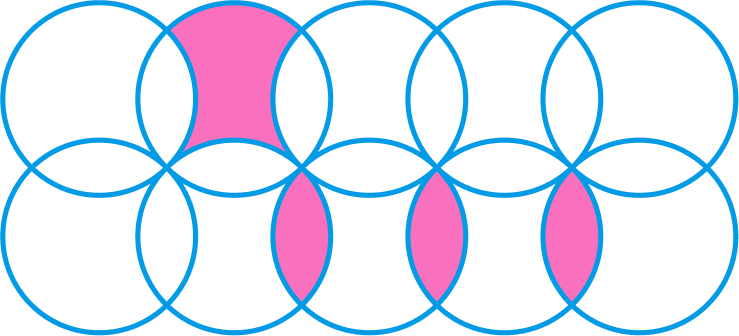# Circular Disposition

Geometry Level 1

Each circle in the diagram below has a radius of $r = 6$. What is the total area of the shaded regions?By this construction, the distance from the center of one circle to its adjoining circles is equal.

×